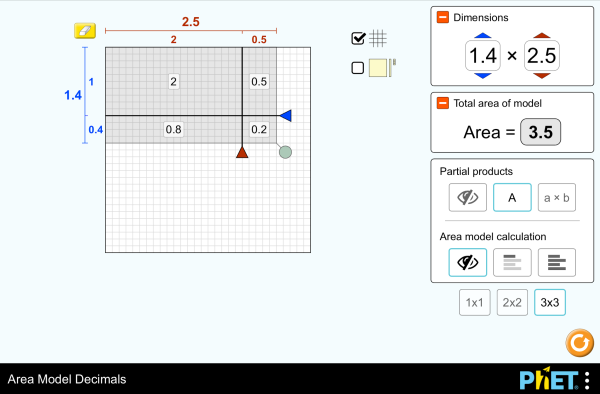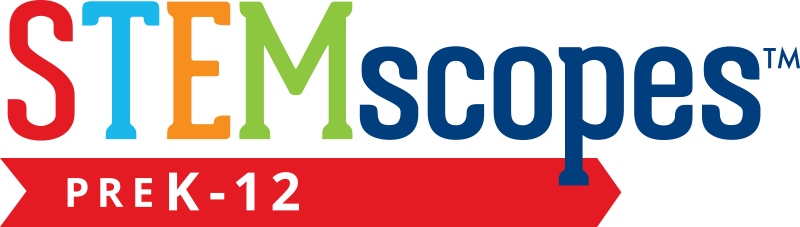# 面積と式の展開（小数）ダウンロード組み込む 閉じる このシミュレーションの実行可能なコピーを組み込む このシミュレーションの実行可能なコピーを組み込むためには、このHTMLコードをお使いください。HTMLコード内の"width"と"height"の属性を変更することによって、組み込まれたシミュレーションの幅と高さを調整できます。 シミュレーション起動用の画像を組み込む
クリックして実行
「クリックして実行」と書かれたスクリーンショットを表示するためには、このHTMLコードをお使いください。 掛け算 Decimals Area Model PhETの支援者あなたの様な教育者の方々

### コンテンツ

• 掛け算
• Decimals
• Area Model
• Factors
• Partial Products

### Description

Build rectangles of various sizes and relate multiplication to area. Partition a rectangle into smaller areas and discover new strategies for multiplying decimals!

### 学習目標例

• Recognize that area represents the product of two numbers and is additive.
• Develop and justify a strategy that uses the area model to simplify a decimal multiplication problem.
• Devise a strategy to determine the product of two rational numbers (or an integer and a rational number) by representing the product as an area or the sum of areas.
• Use an area model to understand why multiplying a given number by a decimal less than 1 results in a product smaller than the given number.
• Interpret the product of two rational numbers using base-ten tiles

### Standards Alignment

#### Common Core - Math

5.NF.B.4
Apply and extend previous understandings of multiplication to multiply a fraction or whole number by a fraction.
5.NF.B.4a
Interpret the product (a/b) × q as a parts of a partition of q into b equal parts; equivalently, as the result of a sequence of operations a × q ÷ b. For example, use a visual fraction model to show (2/3) × 4 = 8/3, and create a story context for this equation. Do the same with (2/3) × (4/5) = 8/15. (In general, (a/b) × (c/d) = ac/bd.)
5.NF.B.4b
Find the area of a rectangle with fractional side lengths by tiling it with unit squares of the appropriate unit fraction side lengths, and show that the area is the same as would be found by multiplying the side lengths. Multiply fractional side lengths to find areas of rectangles, and represent fraction products as rectangular areas.
5.NF.B.5
Interpret multiplication as scaling (resizing), by:
5.NF.B.5a
Comparing the size of a product to the size of one factor on the basis of the size of the other factor, without performing the indicated multiplication.
5.NF.B.5b
Explaining why multiplying a given number by a fraction greater than 1 results in a product greater than the given number (recognizing multiplication by whole numbers greater than 1 as a familiar case); explaining why multiplying a given number by a fraction less than 1 results in a product smaller than the given number; and relating the principle of fraction equivalence a/b = (n × a)/(n × b) to the effect of multiplying a/b by 1.
Version 1.2.1

### Teacher-Submitted Activities

Games Remote Lesson ideasTrish Loeblein 大学基礎

Remote

Area Model Decimals - Grade 6Maura Dudley 中学 Guided 数学
PRIMARIA: Alineación con programas de la SEP México (2011 y 2017)Diana López 小学

Discuss

Guided

Middle School Math Sim AlignmentAmanda McGarry 中学 その他 数学

アラビア語 All العربيةنموذج مساحة الأعداد العشرية
アルバニア語 All shqipModeli i Syprinës: Decimal
イタリア語 All italianoRappresentazione grafica del prodotto di numeri decimali
ウクライナ語 All українськаПлощинна модель десяткових дробів
オランダ語 All NederlandsOppervlakte model decimalen
カザフ語 All KazakhАуданды ондық бөлшектермен есептеу
ギリシャ語 All ΕλληνικάΥπολογισμός εμβαδού - Δεκαδικοί
グジャラート語 All Gujaratiક્ષેત્રફળનું મોડેલ - દશાંશ
シンハラ語 All Sinhaleseවර්ගඑල ආකෘති දශම
スウェーデン語 All svenskaAreamodellen och decimaltal
スペイン語 All españolModelo de Áreas: Decimales
スペイン語（ペルー） All español (Perú)Modelo de área con decimales
スペイン語（メキシコ） All español (México)Modelo de Áreas: Decimales
セルビア語 All СрпскиМатематика преко површина-Децимални бројеви
タミル語 All Tamilமாதிரிகளின் தசமப் பரப்பளவு
デンマーク語 All DanskArealmodel, decimaler
ドイツ語 All DeutschFlächenmodell Dezimalzahlen
トルコ語 All TürkçeAlan Modelinde Çarpma İşlemi (Ondalık)
バスク語 All EuskaraAzalera-modeloa hamartarrekin
ハンガリー語 All magyarTerületmodell: tizedesjegyek
ヒンディー語 All हिंदीनमूने का क्षेत्रफल - दाशमिक
フィンランド語 All suomiPinta-alamalli desimaaleilla
ブラジルポルトガル語 All português (Brasil)Modelo de Área: Decimais
フランス語 All françaisModélisation par les aires avec décimales
ベトナム語 All Tiếng ViệtSố thập phân với mô hình diện tích
ペルシャ語 All فارسیمدل مساحت اعشاری ها
ボスニア語 All BosanskiMatematički model površina - Decimalni brojevi
ポーランド語 All polskiModel pola - ułamki dziesiętne
モンゴル語 All Монгол (Монгол)Талбайн Загвар Бутархайгаар
ラトビア語 All LatviešuLaukuma modeļa decimāldaļskaitļi
ロシア語 All русскийВычисление площади с десятичными дробями

HTML5 sims can run on iPads and Chromebooks, as well as PC, Mac, and Linux systems.

iOS 12+ Safari

Android:
Not officially supported. If you are using the HTML5 sims on Android, we recommend using the latest version of Google Chrome.

Chromebook:
The HTML5 and Flash PhET sims are supported on all Chromebooks.
Chromebook compatible sims

Windows Systems:

Macintosh Systems:

Linux Systems:

デザインチーム サードパーティのライブラリ 謝辞
• Jonathan Olson (developer)
• Karina Hensberry
• Susan Miller
• Ariel Paul
• Kathy Perkins
• almond-0.2.9.js
• base64-js-1.2.0.js
• FileSaver-b8054a2.js
• font-awesome-4.5.0
• game-up-camera-1.0.0.js
• he-1.1.1.js
• himalaya-0.2.7.js
• jama-1.0.2
• jquery-2.1.0.js
• lodash-4.17.4.js
• pegjs-0.7.0.js
• seedrandom-2.4.2.js
• text-2.0.12.js
• TextEncoderLite-3c9f6f0.js